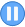﻿

## GMAT Practice Question Set #2 (Question 4-6)

Data Sufficiency Question #4: Triangles Inside Triangles
 Time 0 0 : 0 0 : 0 0In triangle ABC, point X is the midpoint of side AC and point Y is the midpoint of side BC. If point R is the midpoint of line segment XC and if point S is the midpoint of line segment YC ,what is the area of triangular region RCS? (1) The area of triangular region ABX is 32. (2) The length of one of the altitudes of triangle ABC is 8. (A)Statement 1 alone is sufficient but statement 2 alone is not sufficient to answer the question asked. (B)Statement 2 alone is sufficient but statement 1 alone is not sufficient to answer the question asked. (C)Both statements 1 and 2 together are sufficient to answer the question but neither statement is sufficient alone. (D)Each statement alone is sufficient to answer the question. (E)Statements 1 and 2 are not sufficient to answer the question asked and additional data is needed to answer the statements.## Video Explanation

 GMATTM is a registered trademark of the Graduate Management Admission CouncilTM. The Graduate Management Admission CouncilTM does not endorse, nor is it affiliated in any way with the owner or any content of this web site.